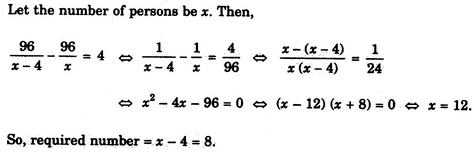# Verbal Reasoning - Arithmetic Reasoning - Discussion

Discussion Forum : Arithmetic Reasoning - Section 1 (Q.No. 4)
4.
A number of friends decided to go on a picnic and planned to spend Rs. 96 on eatables. Four of them, however, did not turn up. As a consequence, the remaining ones had to contribute Rs. 4 each extra. The number of those who attended the picnic was
8
12
16
24
Explanation:Discussion:
45 comments Page 1 of 5.

Karina Nunach said:   10 months ago
Let option A, that 8 students joined picnic and the eatable price is 96Rs. According to question, 4 of them didn't turn up, so the total no of the remaining students are 4, if we divide 96/4=24 rs.

If we contribute this money to 4 students it will be 96 which is the total price, hence option A is correct.
(1)

Naresh Gyawali said:   2 years ago
Check 24 * 4(four extra pay per person) = 96.
96-96=0.

Check 16*4=64
96-64=32 is not the square root.

Check 12*4=48
96-48=48 is not the square root.

Check 8*4=32
96-32=64 is square of 8.

That's why the answer is 8.
(1)

12 * 8 = 96
No of students = 12.
4 students did not turn up, So,
12 - 4 = 8.
(1)

Swathy said:   3 years ago
Right, Agree @Naki.

Rajesh said:   3 years ago
It has 2 answers both A&B.

You can go in two steps:

1. By evaluating through answers (Choices) given. Like you can cross-check those options wise.

8*12 = 96.
4*16 = 96.

OR,

12*8 = 96.
8*12 = 96.

2. Or Answer given in Explanation.

(X-12) (X+8) =0.

Then If X = 12 or X = I8I (+8).

Shivani said:   4 years ago
I think 12 is the answer.

Sankar said:   4 years ago
Total 12 members * Rs. 8 = Rs. 96/-.

If total becomes 8 members (since 4 of them left) * (Rs. 8+extra Rs4/-) = Rs. 96/-.

(1)

Naveen said:   5 years ago
In my point of view.
Total investment =96,
T. A=96/4=24.

4 people don't participated=4*4=16,
Since 24-16=8.

Can we apply this method?
(2)

NAKI said:   5 years ago
Let x bw no of students.

4 absent--> (x-4) students
each student spends rs 4 extra ---> (x-4)*4 for total amount 96rs ---->> (x-4)*4=96 --->> x=28rs.

4 absent.

Hence, 4rs extra ----> 4*4=16rs ------->> x=24-16=12 rs for each who attended.

Now 96/12= 8 students.